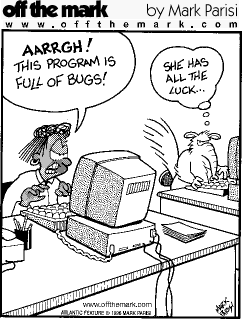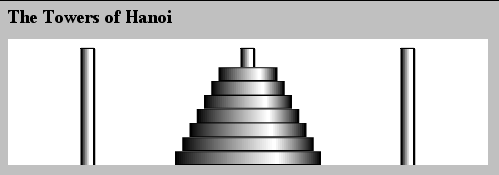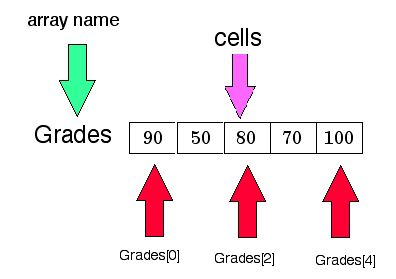CX 103: Introduction to Computers

Lecture 21Date of Second Exam: Tuesday, April 17

Tomorrow's Lab:

JavaScript Questions

Discussion of Fire in the Valley

Exam 1 20%

Exam 2 20%

Final Exam 40%

Poll Results

 First Choice Second Choice Third Choice I: All Quizzes 1 II. Quizzes + HW 3 22 III. HW (all equal) 19 5 1 IV. HW 5%/15% 8 18 1

RECURSION

1) Write a recursive function power( base, exponent) that when invoked returns

baseexponent

Recursive Approach

Terminating Step: base1= base

Recursive Step: baseexponent =base* base(exponent-1)

JavaScript Implementation

function Power(base,exponent)

{ if (exponent == 1) return base;

else return base*Power(base,exponent-1);

}

The Full Script

<HTML>

<TITLE>Recursive Power Function </TITLE>

<SCRIPT LANGUAGE = "Javascript">

function FindPower() {

var base, exponent;

base = document.RecursivePower.base.value;

exponent = document.RecursivePower.exponent.value;

document.RecursivePower.result.value = Power(base,exponent);

function Power(base,exponent)

{ if (exponent == 1) return base;

else return base*Power(base,exponent-1);

}

}

</SCRIPT>

<BODY BGCOLOR = "white">

<H2>

This Program asks you for the <EM>base</EM> and the <EM>exponent</EM> and calculates the base raised to the exponent.

<BR>

<FORM NAME = "RecursivePower">

Enter the base:

<INPUT NAME = "base" TYPE = "text" SIZE = "15">

<BR>

Enter the exponent:

<INPUT NAME = "exponent" TYPE = "text" SIZE = "15">

<BR>

<INPUT TYPE = "button" VALUE = "Submit Numbers" SIZE = "20"

ONCLICK = "FindPower()" >

<BR>

<INPUT NAME = "result" TYPE = "text" SIZE = "30">

</FORM>

</BODY>

</HTML>

Forms

A better way to interact with the user:

Puts all the information on one screen.

Nothing disappears.

Allows program to collect several different pieces of information at once.

We can collect larger pieces of information.

Another Application of Recursion

Converting Decimal to Binary

<HTML>

<TITLE>Converting From Decimal to Binary </TITLE>

<SCRIPT LANGUAGE = "JavaScript">

N = parseInt(window.prompt("Enter N", "") );

Convert(N);

function Convert(N)

{

if (N != 0)

{ Convert(Math.floor(N/2) );

document.write(N % 2) ; }

}

</SCRIPT>

<BODY BGCOLOR = "white"> </BODY>

</HTML>

<HTML>

<TITLE>Convert From Decimal to Arbitrary Base</TITLE>

<SCRIPT LANGUAGE = "JavaScript">

N = parseInt(window.prompt("Enter number to convert from base 10 ", "") );

Base =parseInt(window.prompt("Enter new Base:", " ") );

document.writeln(N + " in base 10 becomes, in base " + Base + " the number " );

Convert(N);

function Convert(N)

{

if (N != 0)

{ Convert(Math.floor(N/Base ));

document.write(N % Base) ; }

}

</SCRIPT>

<BODY BGCOLOR = "white"> </BODY>

</HTML>

Towers of HanoiTowersOfHanoi.html

Assignment 20

3) Write a recursive function which takes an integer value and returns the number with the digits reversed. For example, given the number 13452, the function should return 25431. Incorporate the function into a script that prompts a value from the user. The script should be able to handle an integer with any number of digits (including one). The user simply inputs the number; the user is not asked to tell the program how many digits are in the number.

Recursive Idea:

Chop off units digit

Reverse the rest

Example : 1983

chop off 3: 1983 reverse 198

chop off 8: 198 reverse 19

chop off 9: 19 reverse 1

chop off 1: 1

function ReverseDigits(N)

{     a = N % 10;

document.write(a);

N = (N - a)/10;

if (N > 9) ReverseDigits( N); else if (N !=0) document.writeln(N);

}

<HTML>

<TITLE>Reverse an Integer </TITLE>

<SCRIPT LANGUAGE = "Javascript">

var Number;

Number = window.prompt("Enter an Integer","");

Number = parseInt(Number);

document.writeln("<H1>The original number is " + Number + "<BR>");

document.writeln("The reversed number is ");

ReverseDigits(Number) ;

function ReverseDigits(N)

{ a = N % 10;

document.write(a);

N = (N - a)/10;

if (N > 9) ReverseDigits( N); else if (N !=0) document.writeln(N);

}

</SCRIPT>

<BODY BGCOLOR = "white">

</BODY>

</HTML>

Arrays

Consider a string in JavaScript.

It is a sequence of characters

W = window.prompt("Enter something", "");

Suppose user types in Middlebury

W = Middlebury

 M i d d l e b u r y W W W W W W W W W W

<HTML>

<TITLE>Arrays </TITLE>

<SCRIPT LANGUAGE = "Javascript">

W= window.prompt("Enter a word","");

document.writeln("<H1>The word you gave me was " + W);

document.writeln("<BR>The individual characters are ");

for (i = 0; i <= 9; i = i + 1)

document.writeln("<BR>" + W[i]);

</SCRIPT>

<BODY BGCOLOR = "white"> </BODY>

</HTML>

What if we don't know the length of the word?

W.length tells us.

<HTML>

<TITLE>Arrays </TITLE>

<SCRIPT LANGUAGE = "Javascript">

W= window.prompt("Enter a word","");

document.writeln("<H1>The word you gave me was " + W);

document.writeln("<BR>The individual characters are ");

for (i = 0; i <= W.length - 1; i = i + 1)

document.writeln("<BR>" + W[i]);

</SCRIPT>

<BODY BGCOLOR = "white">

</BODY>

</HTML>

Writing a string backwards

<HTML>

<TITLE>Arrays </TITLE>

<SCRIPT LANGUAGE = "Javascript">

W= window.prompt("Enter a word","");

document.writeln("<H1>The word you gave me was " + W);

document.writeln("<BR><BR>Now we will write it backwards:<BR>");

for (i = W.length-1; i >= 0; i = i - 1)

document.write(W[i]);

</SCRIPT>

<BODY BGCOLOR = "white">

</BODY>

</HTML>

ARRAYS

An array is a collection of objects, each of the same type. The entire collection is referred to using the array name. The items in the array are stored in the array cells and known as elements of the array. The cells are referenced using the array index or subscript.

In JavaScript (as well as Java, C and C++), all array subscripts start with 0.

Example: the five exam scores 90, 50, 80, 70, 100 can be stored in an array called "Grades" which we can picture asNumber of cells = 5

Range of index = 0 to 4

name of third cell = Grades

The syntax for "declaring" such an array:The declaration only sets up space in memory for the array.

We must "initialize" with reliable values before they can be used.

Method I:

var Grades = new Array( 5 );

Here are some other ways:

var Grades = new Array (90, 50, 80, 70, 100);

var Grades = [90, 50, 80, 70, 100];

Grades = [90, 50, 80, 70, 100];

<HTML>

<TITLE> Initializing Arrays </TITLE>

<SCRIPT LANGUAGE = "JavaScript">

var i;

var Grades = new Array (90, 50, 80, 70, 100);

GradeString = "var Grades = new Array (90, 50, 80, 70, 100);"

for (i = 0; i <= 4; i = i+1) document.writeln( Grades[i] );

document.writeln("<BR><BR>");

var Scores = [90, 50, 80, 70, 100];

ScoresString = "var Scores = [90, 50, 80, 70, 100];"

document.writeln("<EM>"+ ScoresString + "</EM><BR>");

document.writeln("The Scores are :");

for (i = 0; i <= 4; i = i+1) document.writeln( Scores[i] );

document.writeln("<BR><BR>");

Results = [90, 50, 80, 70, 100];

ResultsString = "Results = [90, 50, 80, 70, 100];"

document.writeln("<EM>"+ ResultsString + "</EM><BR>");

document.writeln("The Results are :");

for (i = 0; i <= 4; i = i+1) document.writeln( Results[i] );

document.writeln("<BR>");

N = window.prompt("How Many Squares", "")

N = parseInt(N);

document.writeln("<BR>Dynamic size array with " + N +" elements:<BR>");

var Exams = new Array(N);

for (i = 0; i <= N-1; i = i+1)

{ Exams[i] = [i];

document.writeln(i*i)

}

document.writeln("<BR><BR><EM>An Array of Strings</EM><BR>")

document.writeln("The Names are: ");

var Names = ["Kate", "Ted", "Trevor", "Homer", "Bob", "Becky"];

for (i = 0; i <= Names.length - 1; i = i + 1) document.writeln( Names[i] );

document.writeln("<BR>");

</SCRIPT>

<BODY BGCOLOR = "blanchedalmond">

</BODY>

</HTML>

Application: Word Counter

<HTML>

<TITLE> Word Counter </TITLE>

<SCRIPT LANGUAGE = "JavaScript">

function DoStuff()

{

text = document.MyForm.phrase.value;

Words = 1;

for( i = 0; i <= text.length - 1; i = i + 1)

if (text[i] == " ") Words = Words+1;

document.MyForm.HowManyWords.value = Words;

}

</SCRIPT>

<BODY BGCOLOR = "white">

<FORM NAME = "MyForm">

<H2>

Enter A Phrase:

<INPUT NAME = "phrase" TYPE = "text" SIZE = "50">

<BR>

<INPUT TYPE = "button" VALUE = "Click to Count" SIZE = "20"

ONCLICK = "DoStuff()" >

<BR>

Number of Words:

<INPUT NAME = "HowManyWords" TYPE = "text" SIZE = "15">

</FORM> </BODY></HTML>

Allow Large Amount of Text

<HTML>

<TITLE> Word Counter for Large Amount of Text </TITLE>

<SCRIPT LANGUAGE = "JavaScript">

function DoStuff()

{

text = document.MyForm.phrase.value;

Words = 1;

for( i = 0; i <= text.length - 1; i = i + 1)

if (text[i] == " ") Words = Words+1;

document.MyForm.HowManyWords.value = Words;

}

</SCRIPT>

<BODY BGCOLOR = "blanchedalmond">

<FORM NAME = "MyForm">

<H2>

Enter Some Text

<TEXTAREA NAME = "phrase" ROWS = 20 COLS = 50> </TEXTAREA>

<BR>

<INPUT TYPE = "button" VALUE = "Click to Count" SIZE = "20"

ONCLICK = "DoStuff()" >

<BR>

Number of Words:

<INPUT NAME = "HowManyWords" TYPE = "text" SIZE = "15">

</FORM>

</BODY>

</HTML>